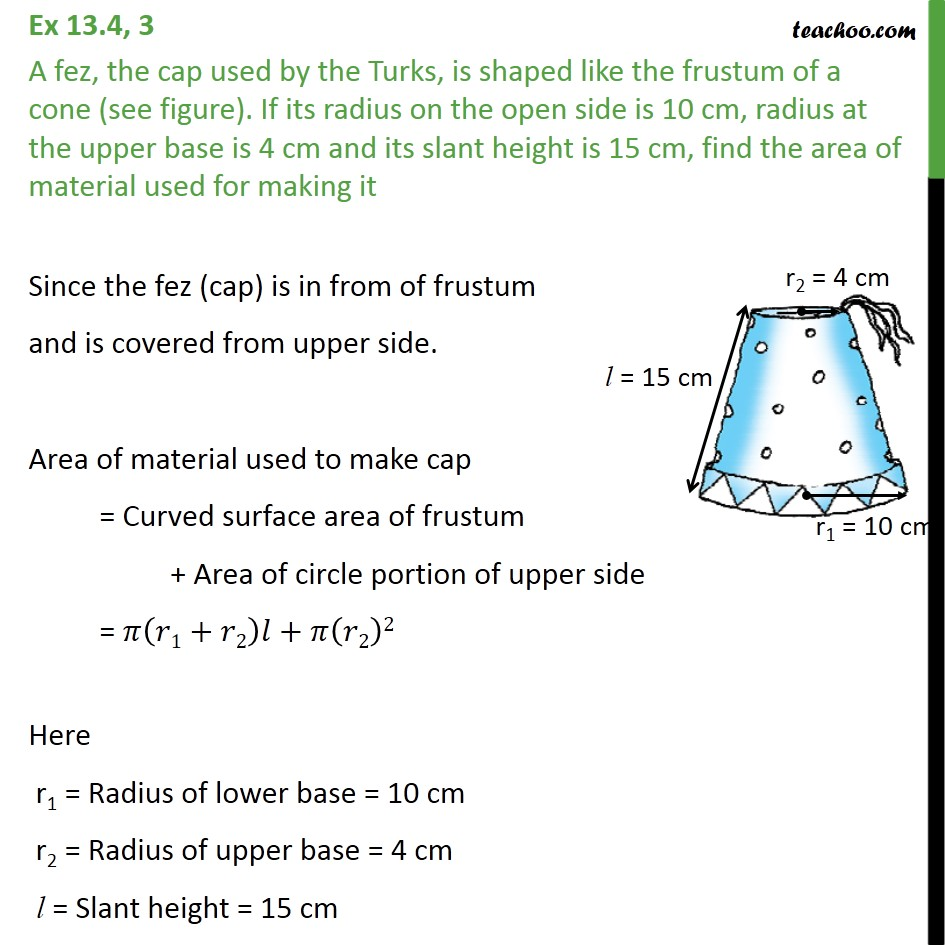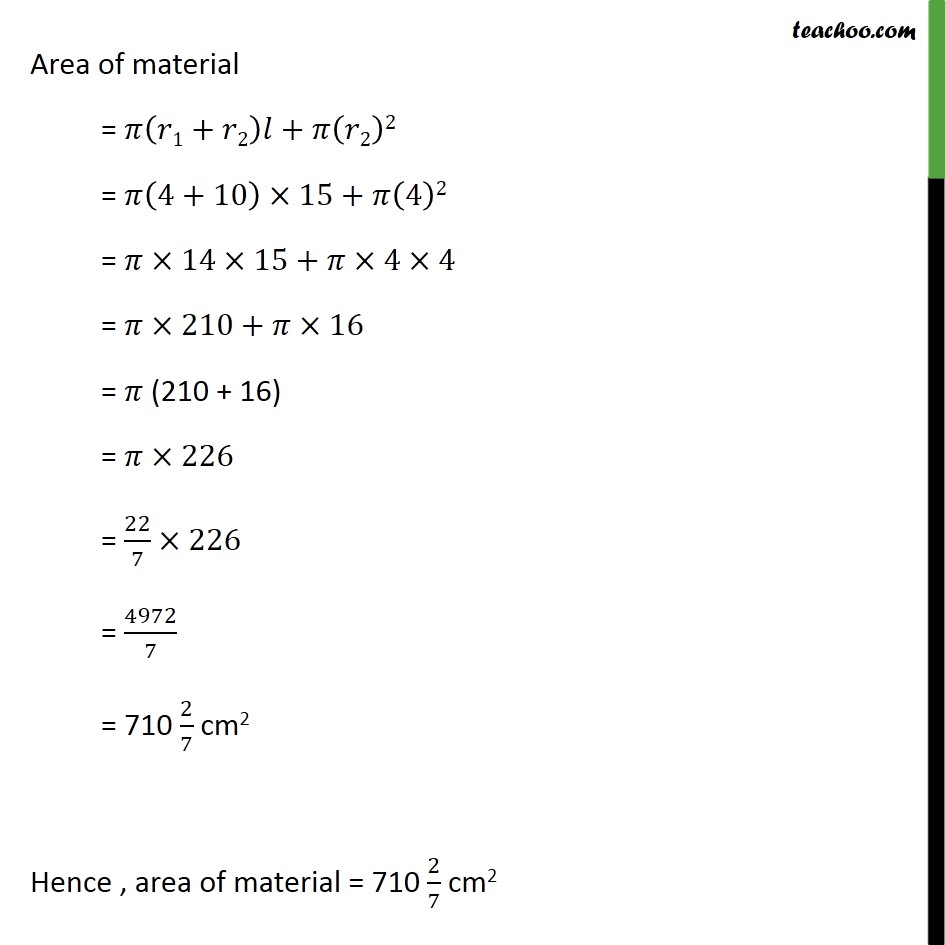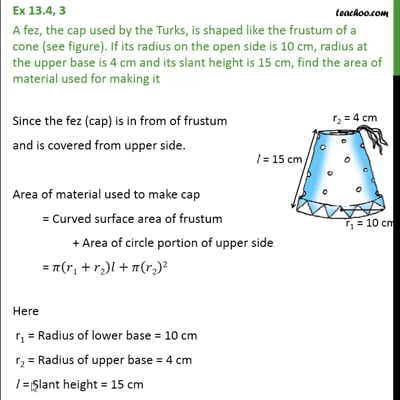Ex 13.4

Chapter 13 Class 10 Surface Areas and Volumes (Term 2)
Serial order wiseThis video is only available for Teachoo black users

### Transcript

Ex 13.4, 3 A fez, the cap used by the Turks, is shaped like the frustum of a cone (see figure). If its radius on the open side is 10 cm, radius at the upper base is 4 cm and its slant height is 15 cm, find the area of material used for making it Since the fez (cap) is in from of frustum and is covered from upper side. Area of material used to make cap = Curved surface area of frustum + Area of circle portion of upper side = 𝜋(𝑟1+𝑟2)𝑙+𝜋(𝑟2)2 Here r1 = Radius of lower base = 10 cm r2 = Radius of upper base = 4 cm l = Slant height = 15 cm Area of material = 𝜋(𝑟1+𝑟2)𝑙+𝜋(𝑟2)2 = 𝜋(4+10)×15+𝜋(4)2 = 𝜋×14×15+𝜋×4×4 = 𝜋×210+𝜋×16 = 𝜋 (210 + 16) = 𝜋×226 = 22/7×226 = 4972/7 = 710 2/7 cm2 Hence , area of material = 710 2/7 cm2